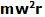## Sunday, February 15, 2009

### Irodov Problem 1.276(a)
The force F acts through the axis of rotation and so does not impart any torque on the rotating (disc + point mass) system. As a consequence the angular momentum of the (disc + point mass system) is always conserved. As the point mass moves radially inwards, this decreases the moment of inertia of the (disc + point mass system) and thus increases the angular velocity.

The moment of inertia of the (disc + point mass) system about the axis of rotation, when the point mass is at a distance r from the center is given by is given by. Since the initial angular velocity of the disc when r=R is w0, since angular momentum is conserved we have,In its final state, r=0 since the point mass reaches the center. Thus, setting r=0 in (1) we have,(b) Since the problem states that the point mass in the radial guide is pulled very slowly, it means that the point mass was not imparted any acceleration. In the rotating reference frame, there are two forces acting on the point mass in the radial direction when it is at a distance r from the center, i) the force F in the thread pulling the point mass radially inwards, and ii) the centrifugal forcepulling the point mass outwards. Since there is no radial acceleration, the force F must exactly balance the centrifugal force. In other words,The work done by the force F is then given by,